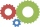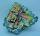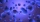# Geometric progression + exponential equation - math problems

#### Number of problems found: 21

• Geometric progressionIn geometric progression, a1 = 7, q = 5. Find the condition for n to sum first n members is: sn≤217.
• Geometric sequenceIn the geometric sequence is a4 = 20 a9 = -160. Calculate the first member a1 and quotient q.
• Six speedsA drilling machine is to have 6 speed ranging from 50 to 750 revolution per minute. If the speed forms a geometric progression, determine their values.
• Geometric sequence 3In geometric sequence is a8 = 312500; a11= 39062500; sn=1953124. Calculate the first item a1, quotient q and n - number of members by their sum s_n.
• Insert into GPBetween numbers 5 and 640 insert as many numbers to form geometric progression so sum of the numbers you entered will be 630. How many numbers you must insert?
• SequenceCalculate what member of the sequence specified by ? has value 86.
• Compound interestCalculate time when deposit in the bank with interest 2.5% p.a. doubles.A radioactive material loses 10% of its mass each year. What proportion will be left there after n=6 years?
• DemographicsThe population grew in the city in 10 years from 30000 to 34000. What is the average annual percentage increase of population?
• Investment1000\$ is invested at 10% compound interest. What factor is the capital multiplied by each year? How much will be there after n=12 years?
• Half lifeDetermine the half life of bismuth, when bismuth weight from the original weight of 32 g was only 2 grams in 242 minutes.
• Growth of woodThe annual growth of wood in the forest is estimated at 2%. In how many years will make the forest volume double?
• Annual growthThe population has grown from 25,000 to 33,600 in 10 years. Calculate what was the average annual population growth in%?
• CrystalThe crystal grows every month 1.2 permille of its mass. For how many months to grow a crystal from weight 177 g to 384 g?
• MicroorganismsThe first generation of micro-organisms has a population of 13500 members. Each next generation is 11/10 times the previous one. Find out how many generations will reach at least three times members of the first generation.
• Volume of woodEvery year, at the same time, an increase in the volume of wood in the forest is measured. The increase is regularly p% compared to the previous year. If in 10 years the volume of wood has increased by 10%, what is the number p?
• To the cinemaJane at 8 am got message that all 1093 school pupils will go to the cinema. Within 20 min she said it to the three friends. Each of them again for 20 minutes said to the other three. In this way the message spread further. At what time all the children in
• Investment 2Jack invested \$5000 in a 5-month term deposit at 4.7% p. A. . At the end of the 5-months, jack reinvested the maturity value from the first deposit into an 11-month term deposit at 7.3% p. A. What is the maturity value at the end of the second term deposi
• SavingsThe depositor regularly wants to invest the same amount of money in the financial institution at the beginning of the year and wants to save 10,000 euros at the end of the tenth year. What amount should he deposit if the annual interest rate for the annua
• Exponential decayA tank contains 55 liters of water. Water is flowing out at the rate of 7% per minute. How long does it take to drain the tank?

Do you have an interesting mathematical word problem that you can't solve it? Submit a math problem, and we can try to solve it.

We will send a solution to your e-mail address. Solved examples are also published here. Please enter the e-mail correctly and check whether you don't have a full mailbox.

Please do not submit problems from current active competitions such as Mathematical Olympiad, correspondence seminars etc...

Geometric progression - math word problems. Exponential equation - math word problems.